• Weather
• Ocean
• Ice & Icebergs
• Other Hazards
• Digital Forecasts
• Electronic Marine Charting (ECDIS)
• Environmental
• Extratropical Storm Surge Models
• Freezing Spray
• GIS
• Observations
• Ocean
• Past Weather
• Probabilistic
• Product Information
• Receiving Products
• News
• Social Media

 Hurricane Warnings in Effect Atlantic:  High Seas

## GEFSWAVE Probabilistic Wave Height Guidance

Use the drop down menus below to change the display.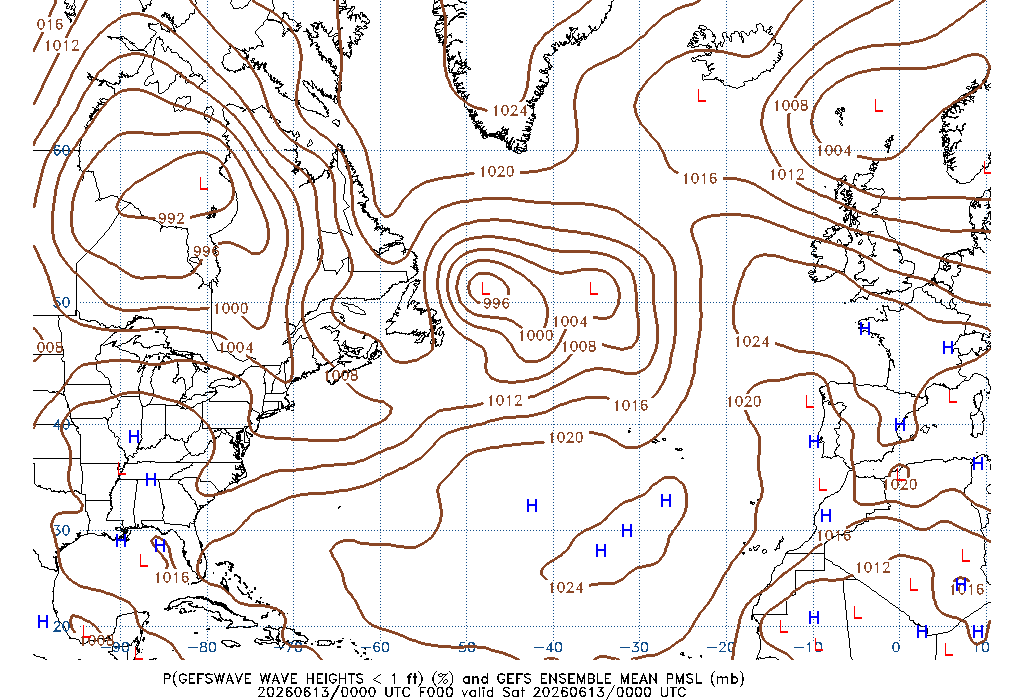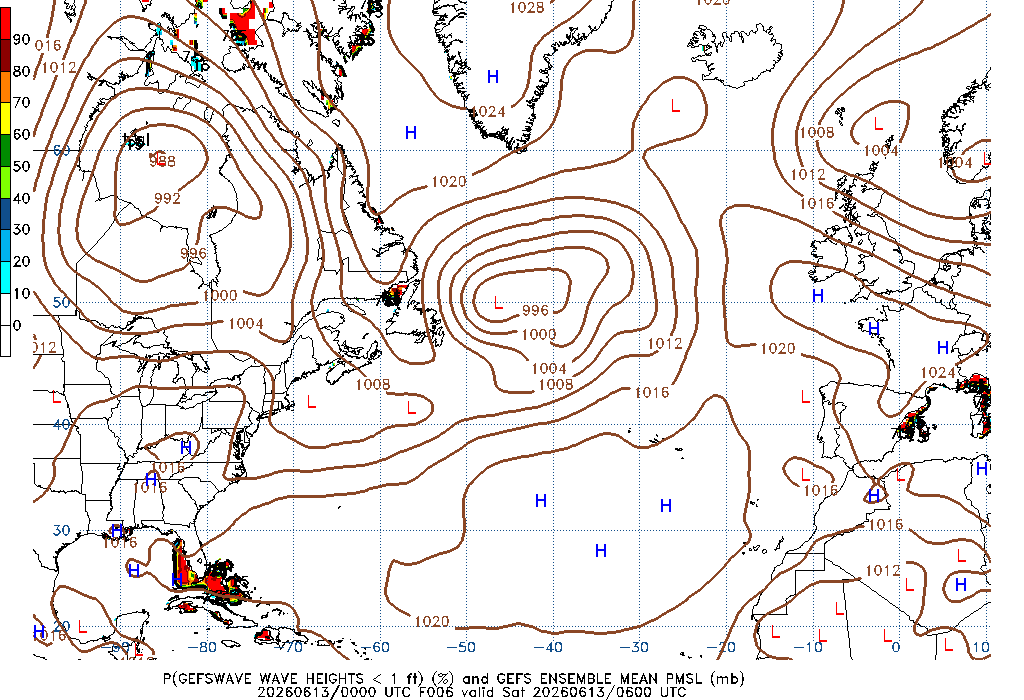000 Hour Wave Height less than 1ft 006 Hour Wave Height less than 1ft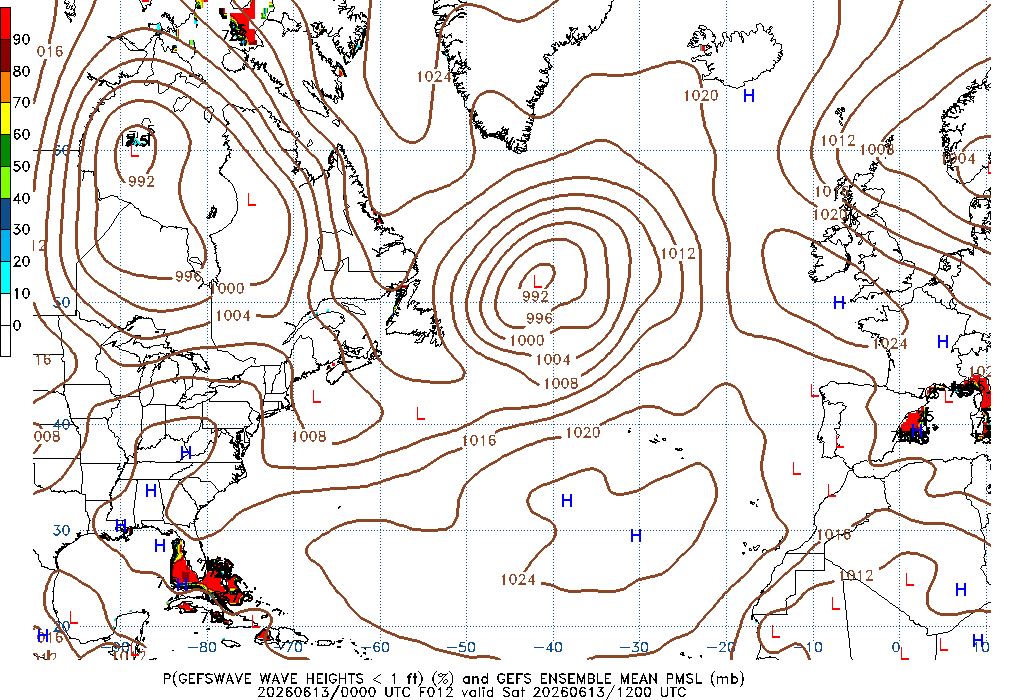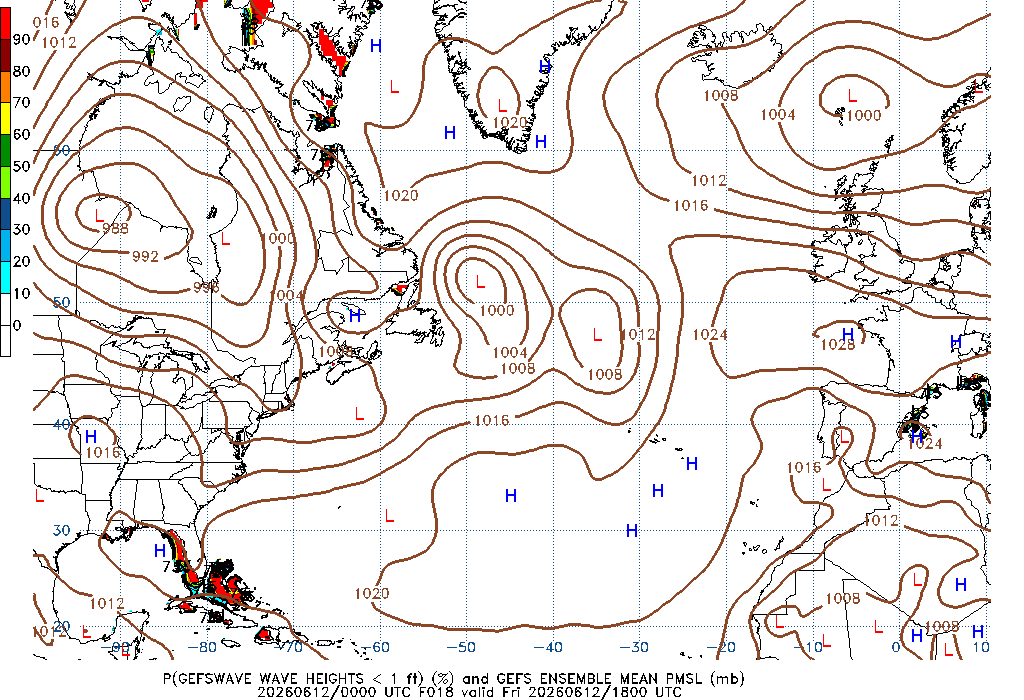012 Hour Wave Height less than 1ft 018 Hour Wave Height less than 1ft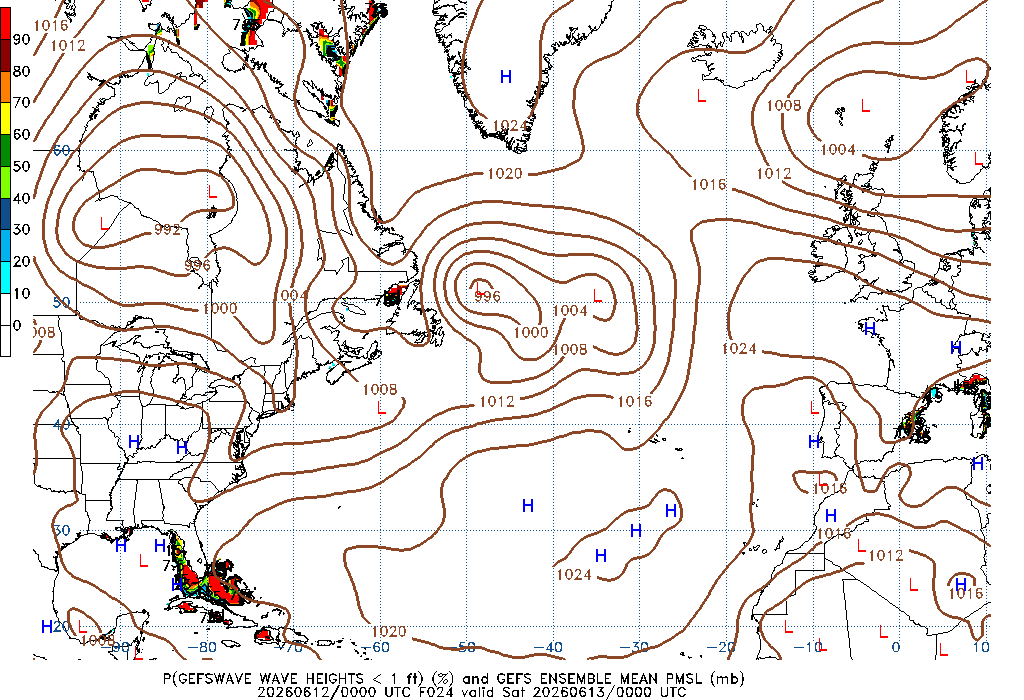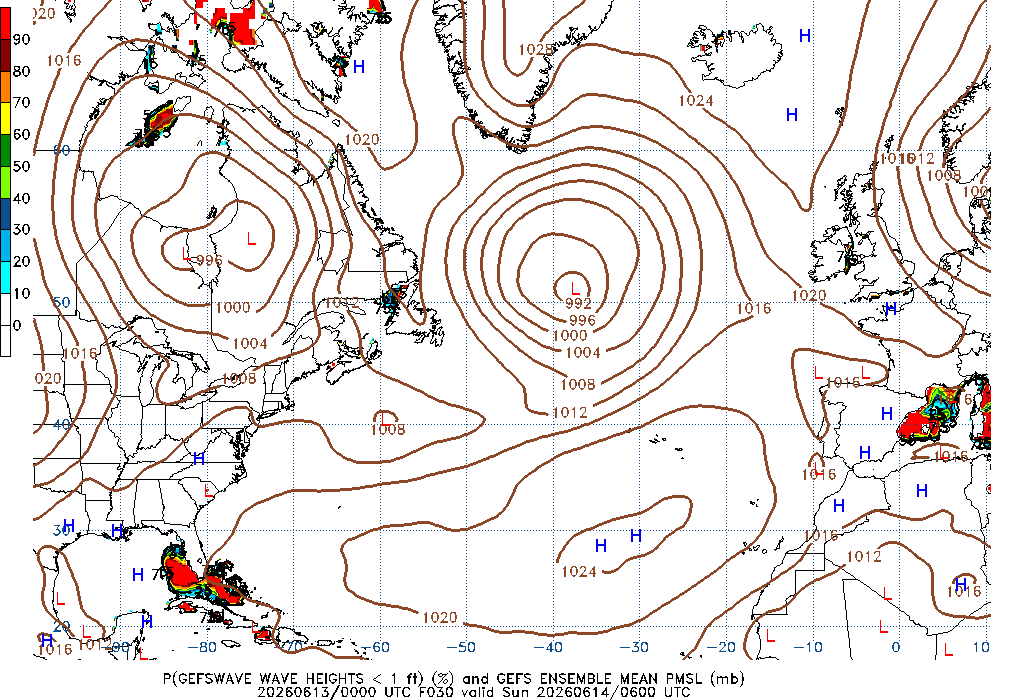024 Hour Wave Height less than 1ft 030 Hour Wave Height less than 1ft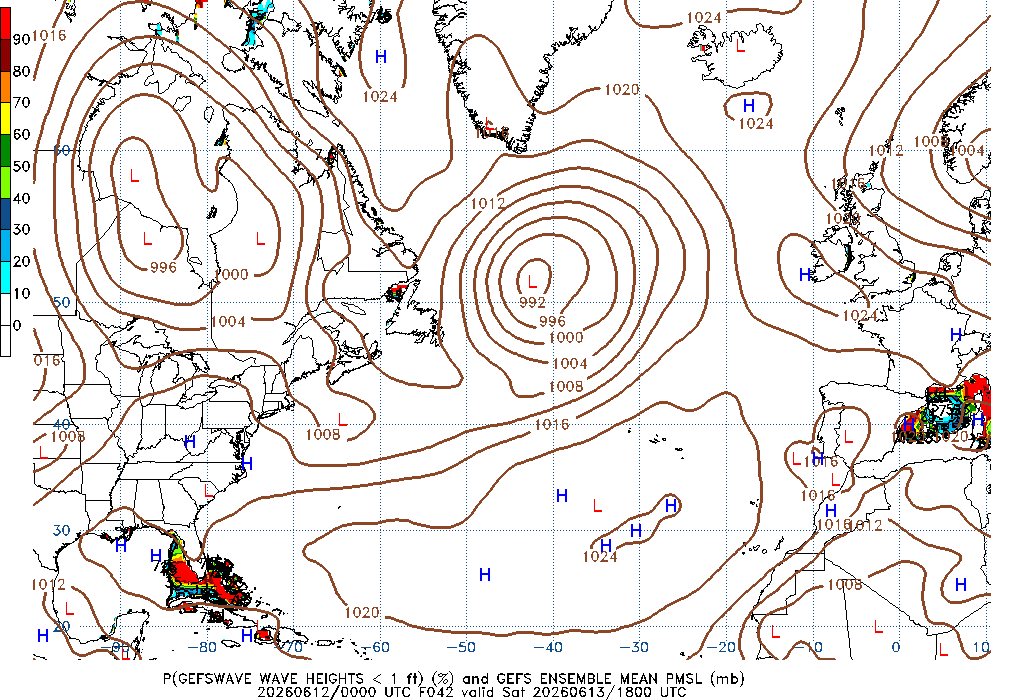036 Hour Wave Height less than 1ft 042 Hour Wave Height less than 1ft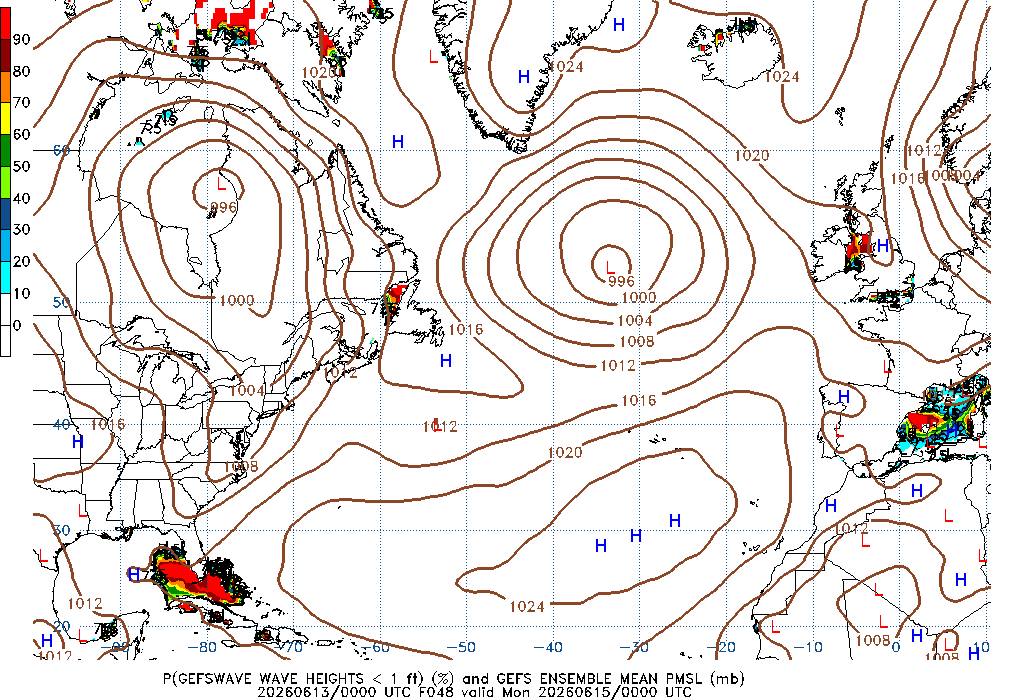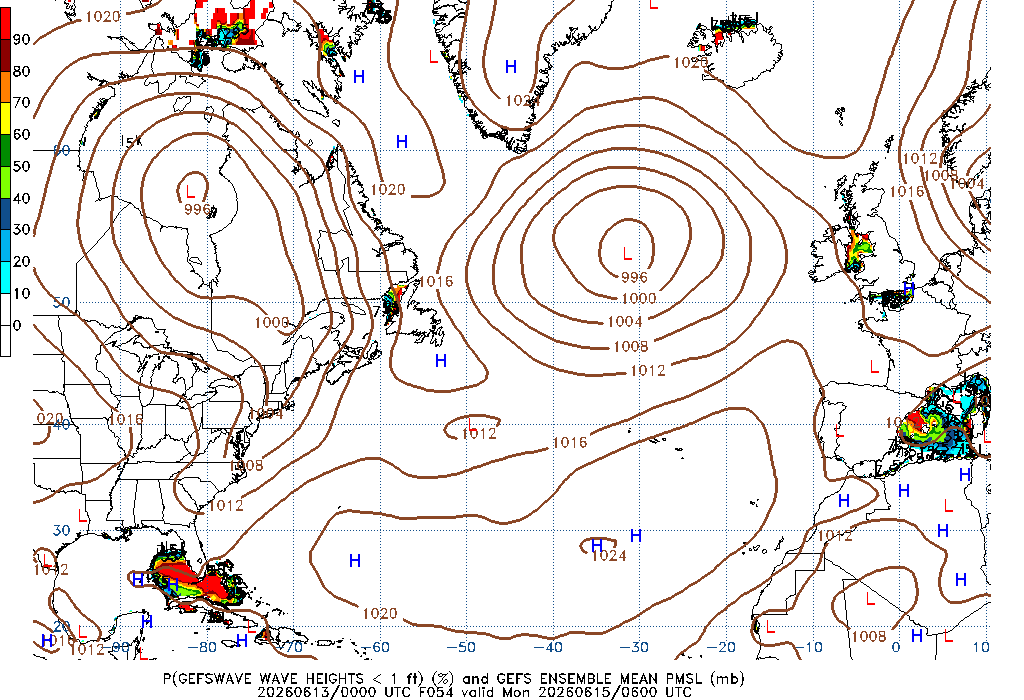048 Hour Wave Height less than 1ft 054 Hour Wave Height less than 1ft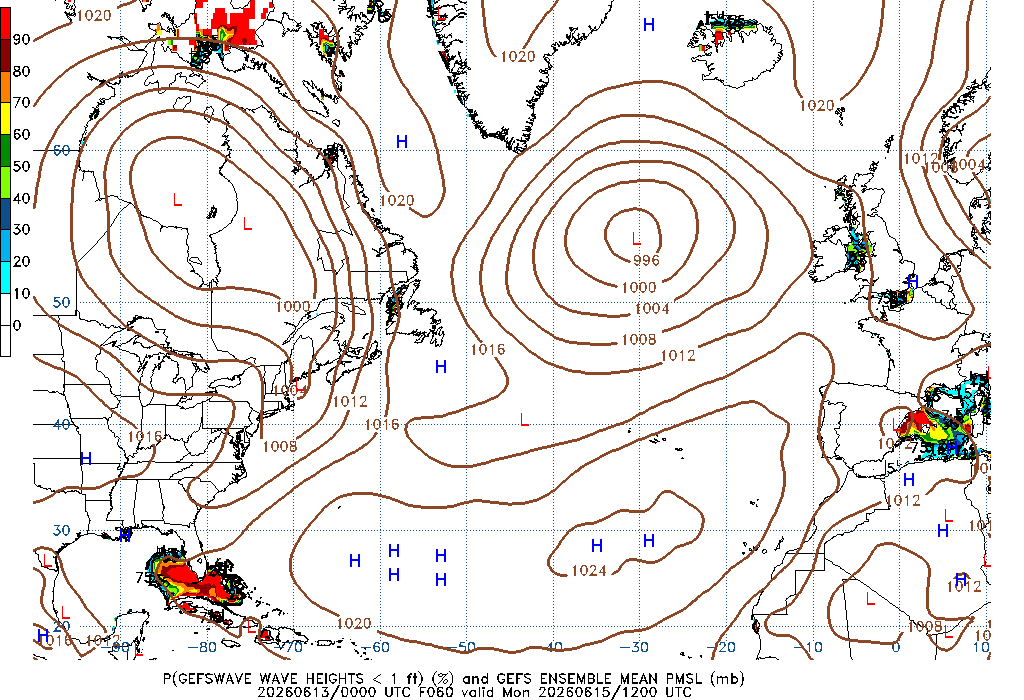060 Hour Wave Height less than 1ft 066 Hour Wave Height less than 1ft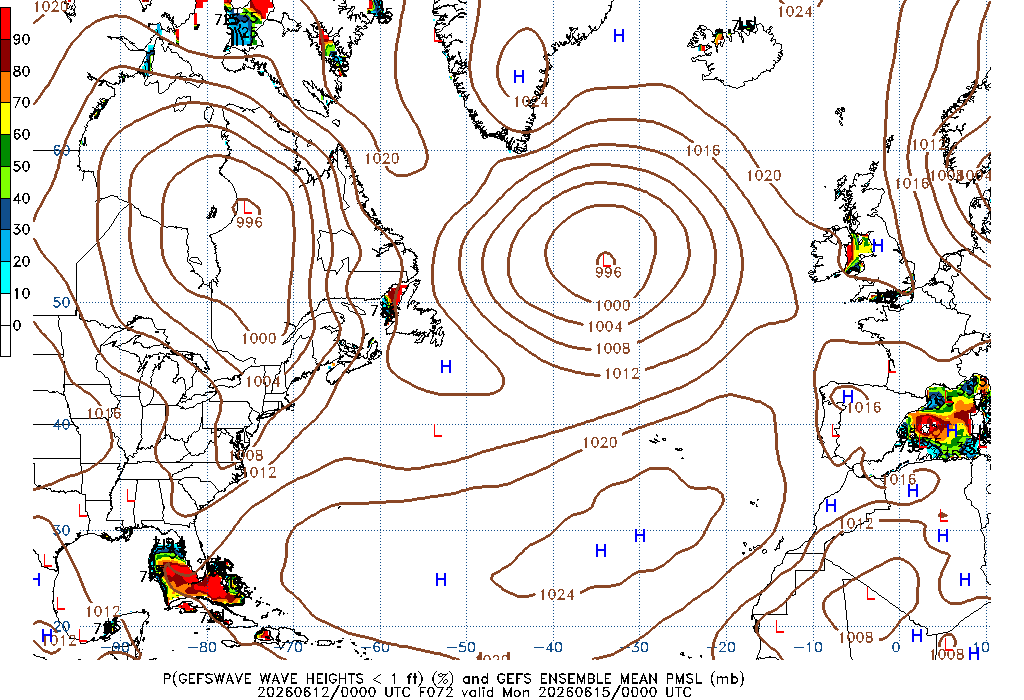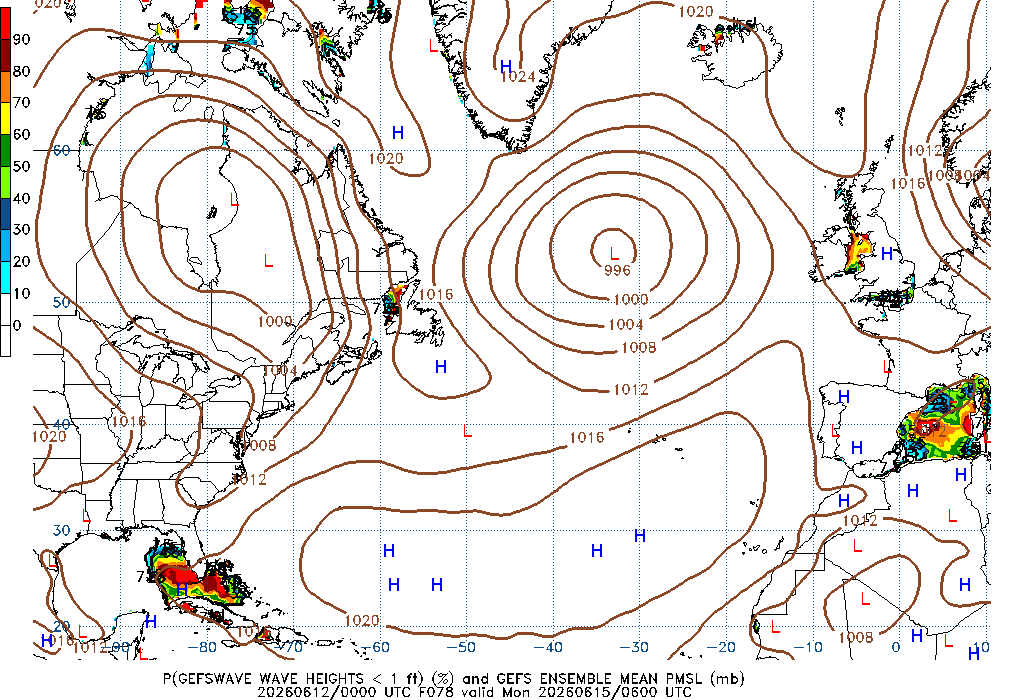072 Hour Wave Height less than 1ft 078 Hour Wave Height less than 1ft084 Hour Wave Height less than 1ft 090 Hour Wave Height less than 1ft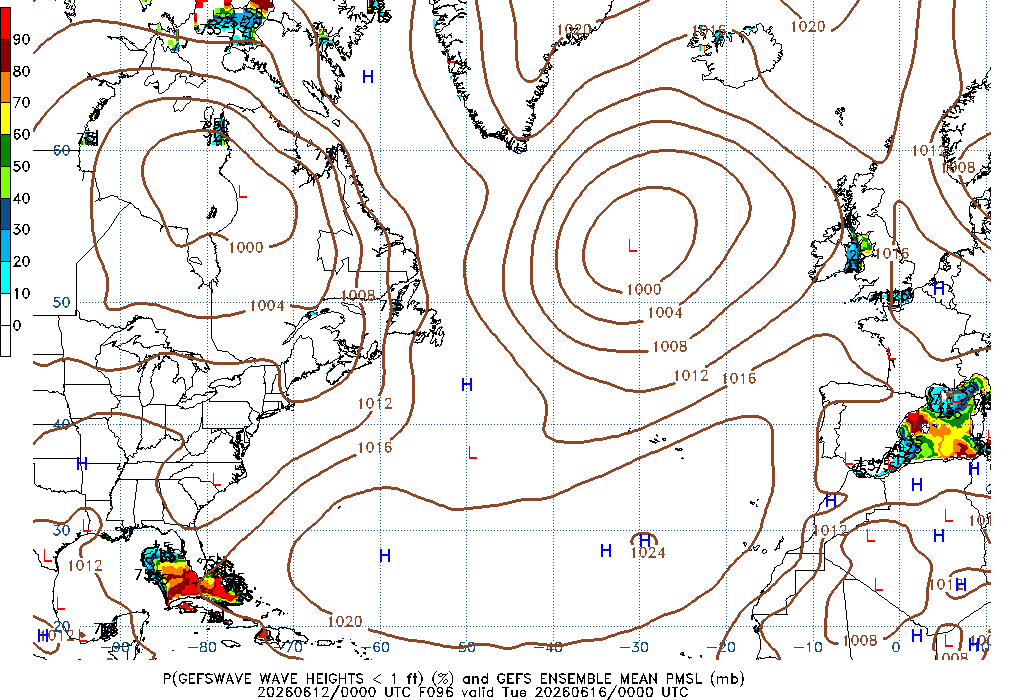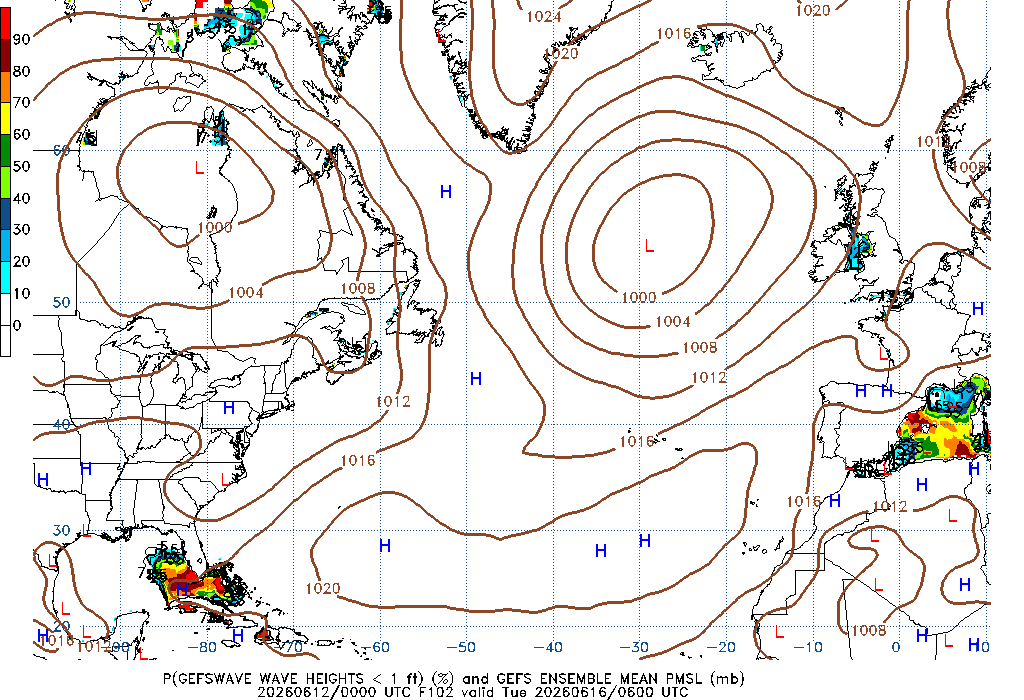096 Hour Wave Height less than 1ft 102 Hour Wave Height less than 1ft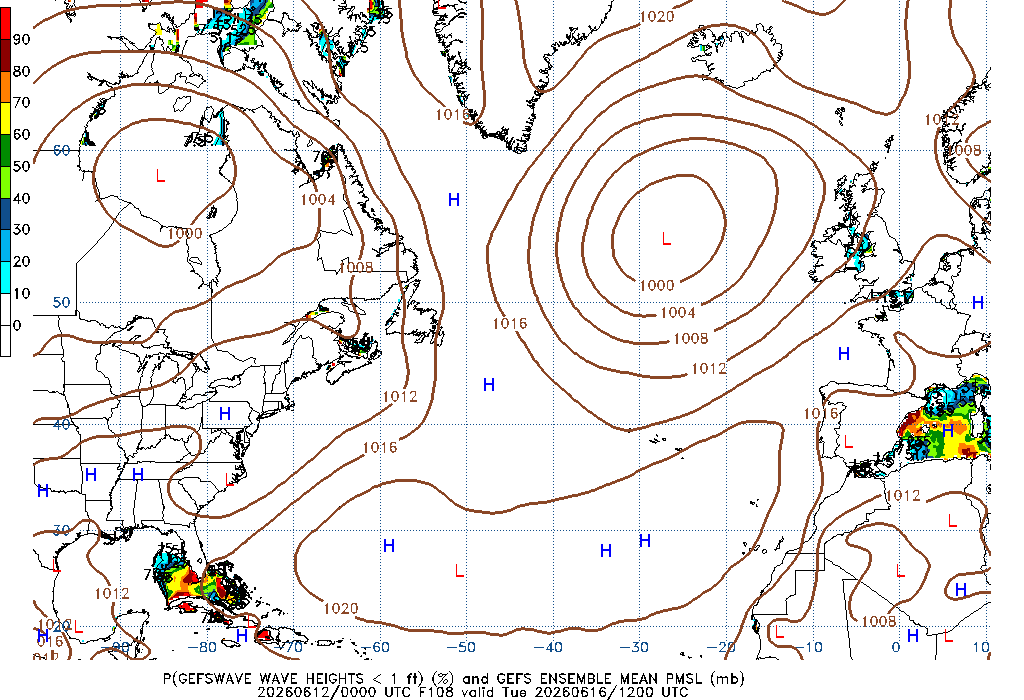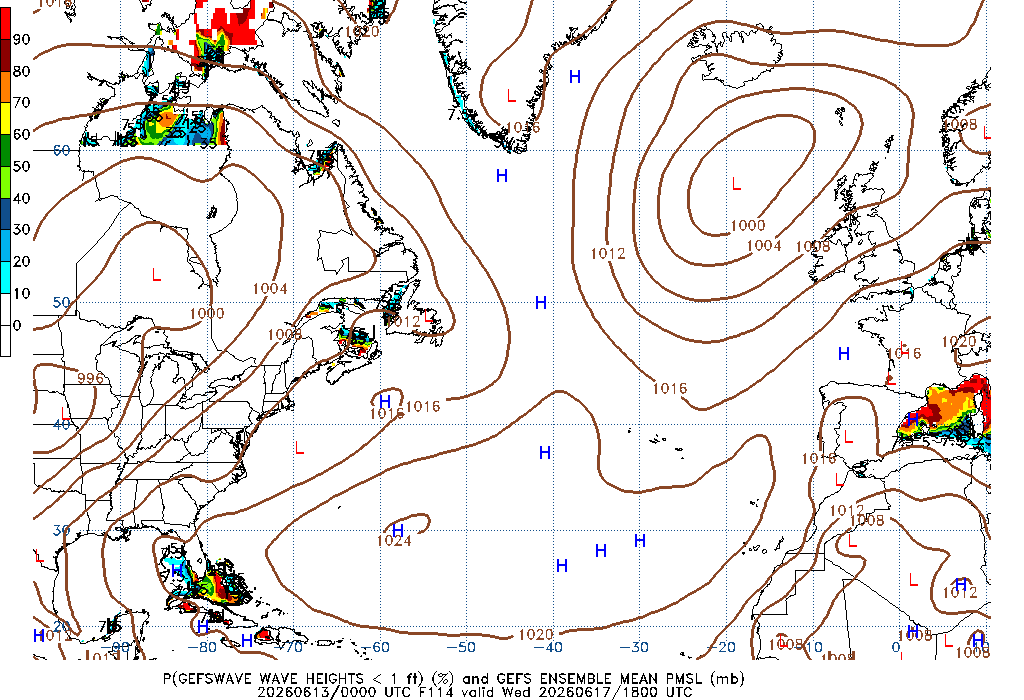108 Hour Wave Height less than 1ft 114 Hour Wave Height less than 1ft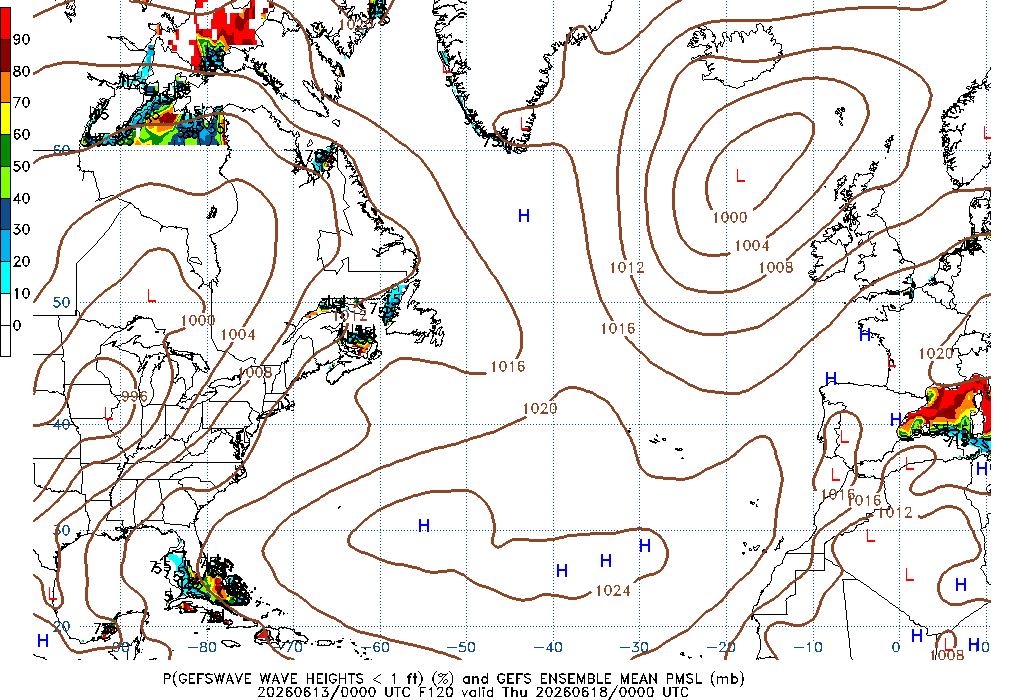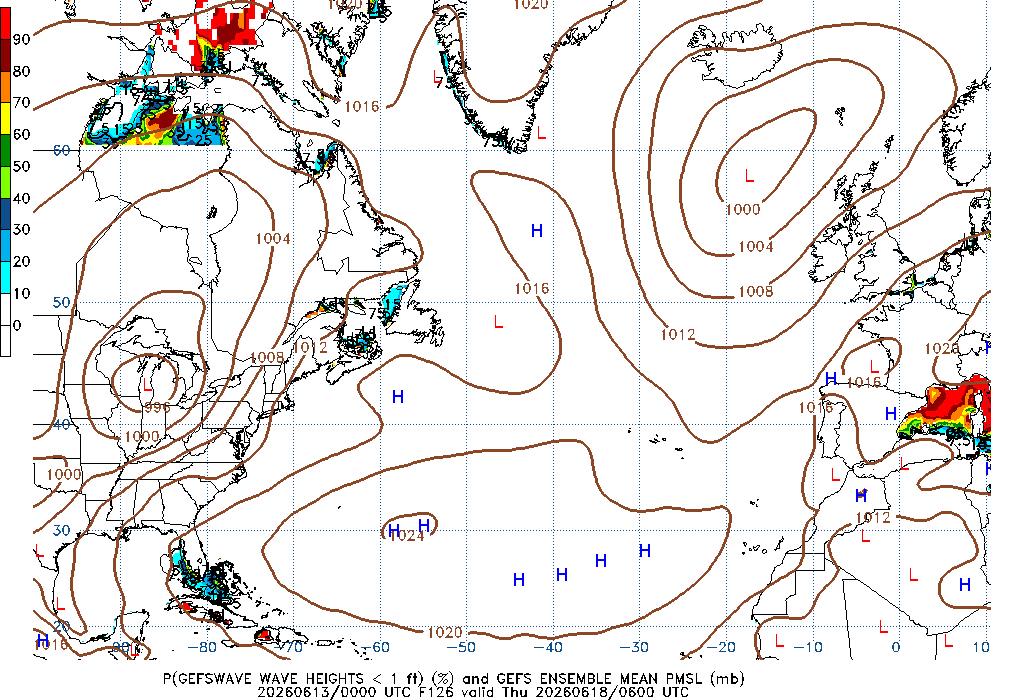120 Hour Wave Height less than 1ft 126 Hour Wave Height less than 1ft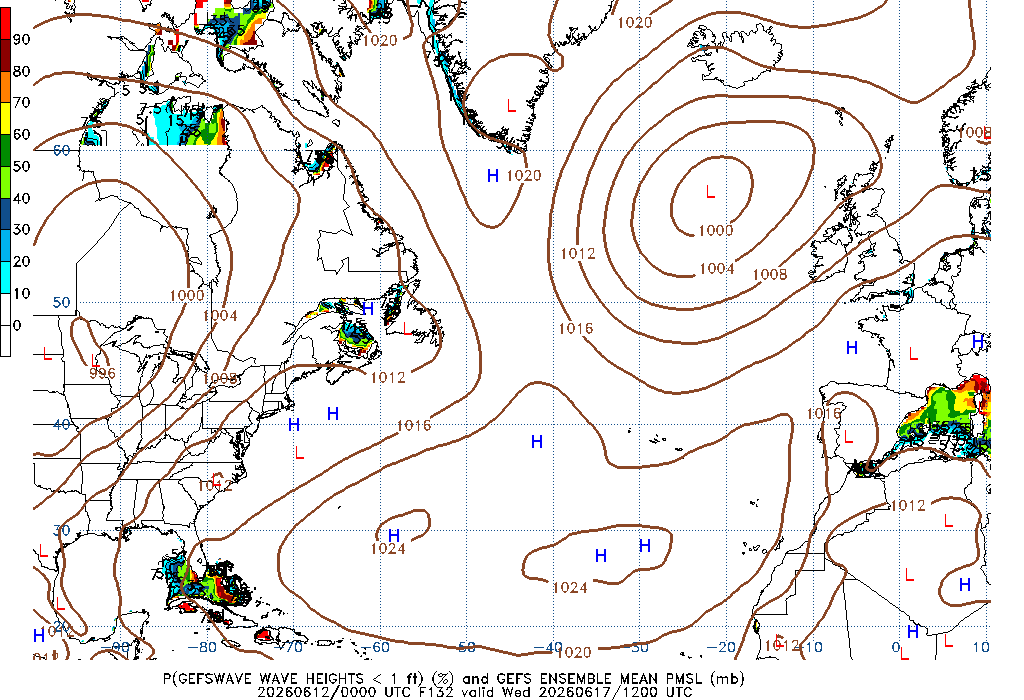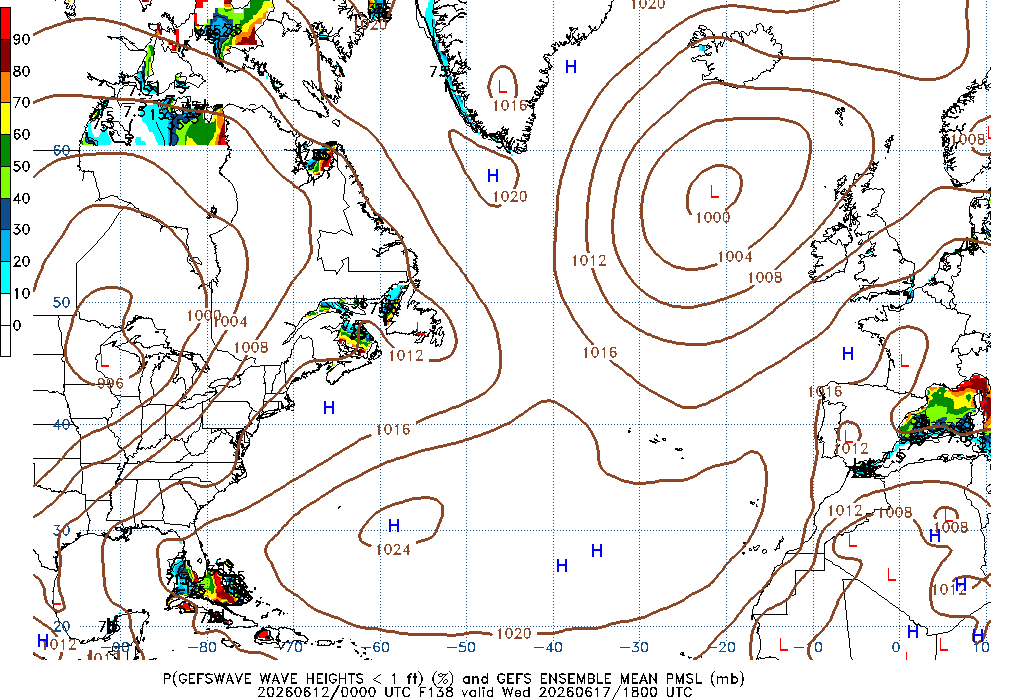132 Hour Wave Height less than 1ft 138 Hour Wave Height less than 1ft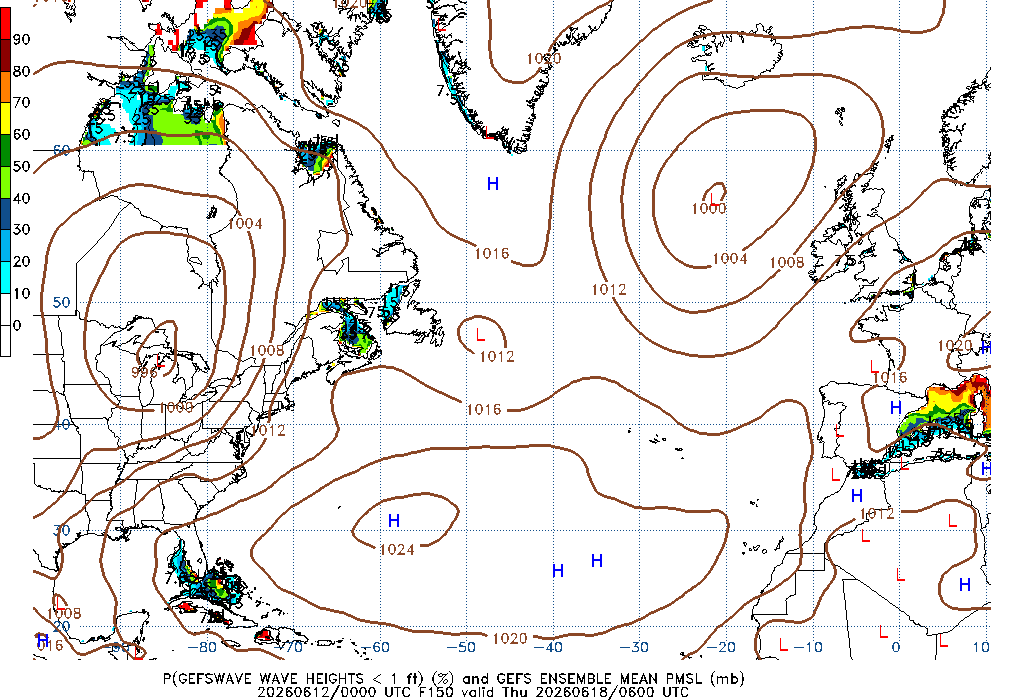144 Hour Wave Height less than 1ft 150 Hour Wave Height less than 1ft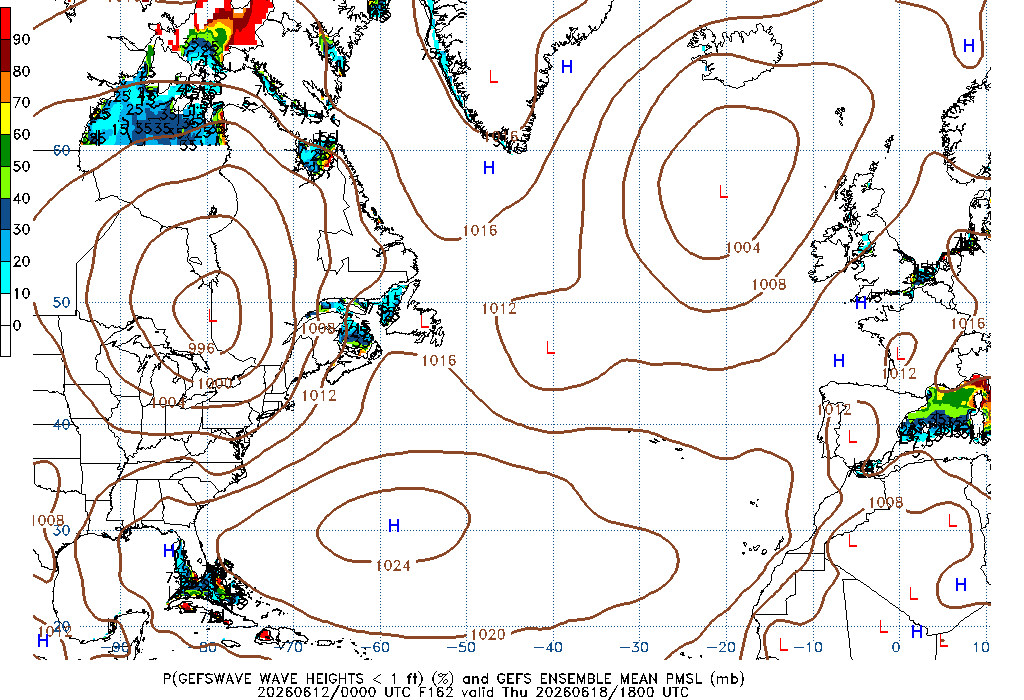156 Hour Wave Height less than 1ft 162 Hour Wave Height less than 1ft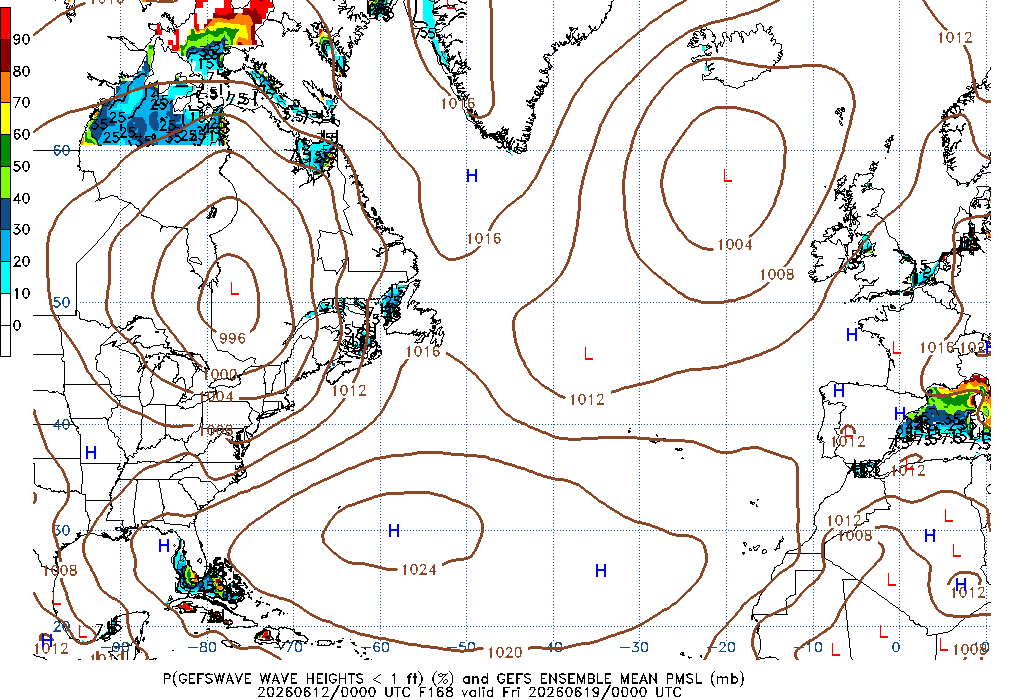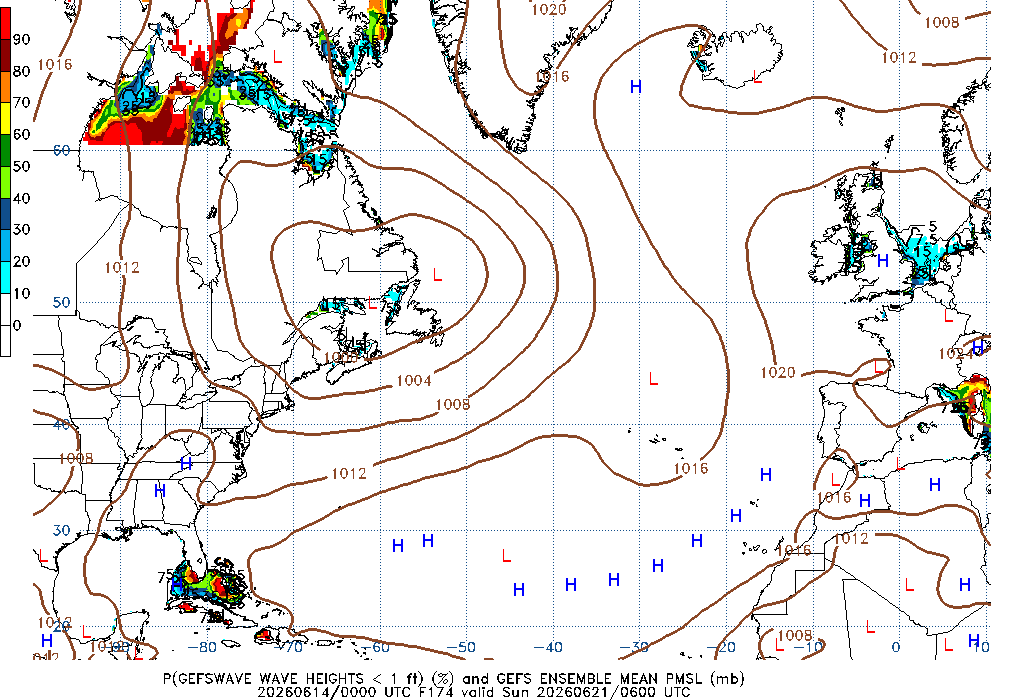168 Hour Wave Height less than 1ft 174 Hour Wave Height less than 1ft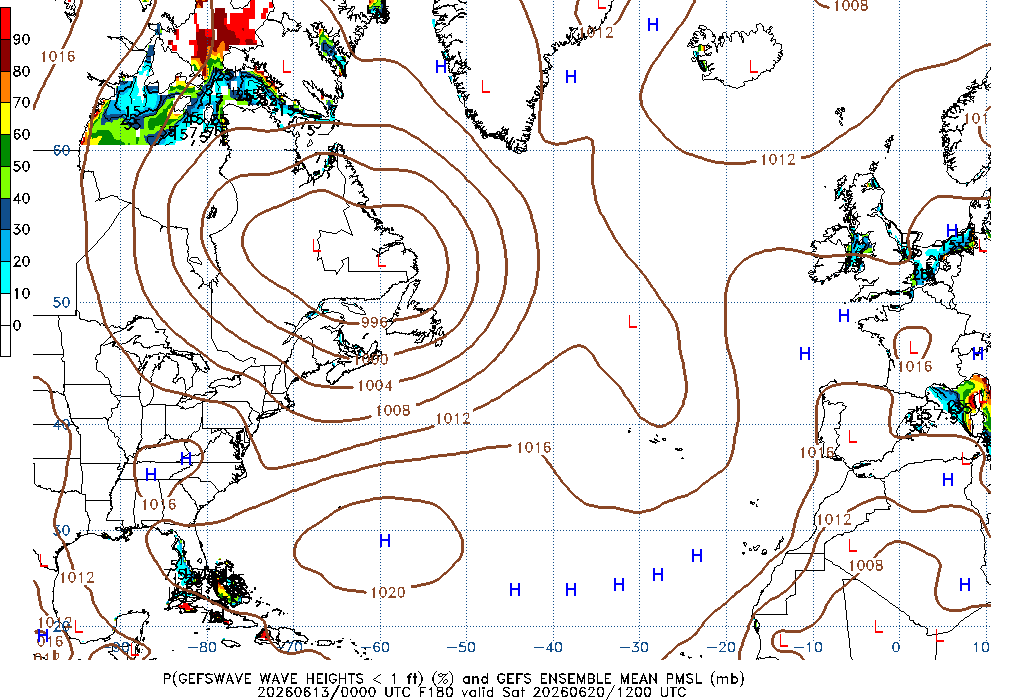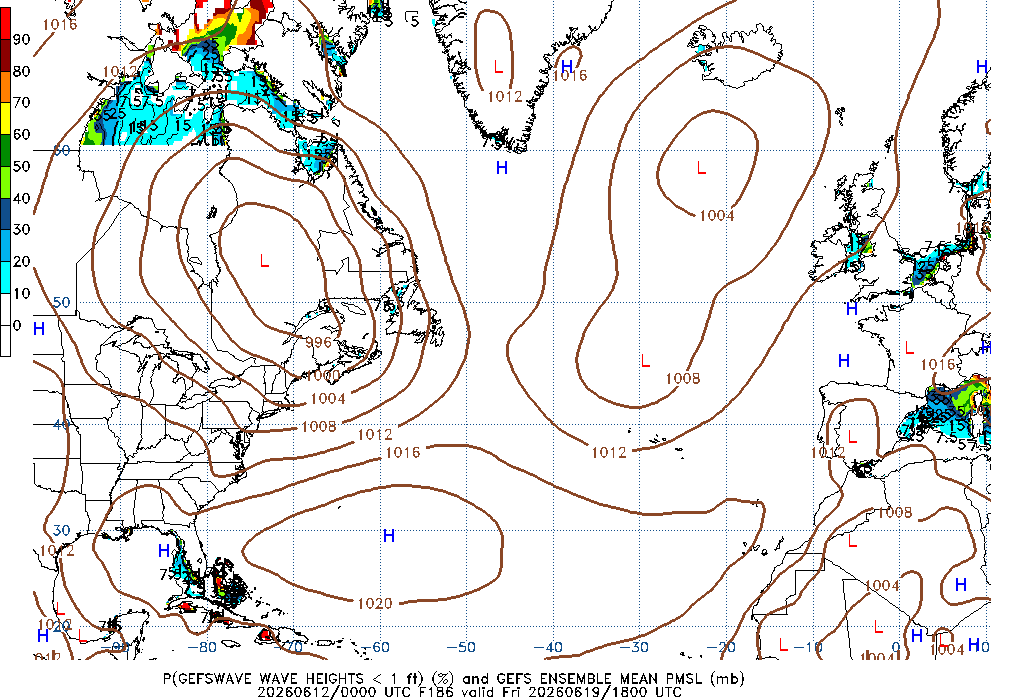180 Hour Wave Height less than 1ft 186 Hour Wave Height less than 1ft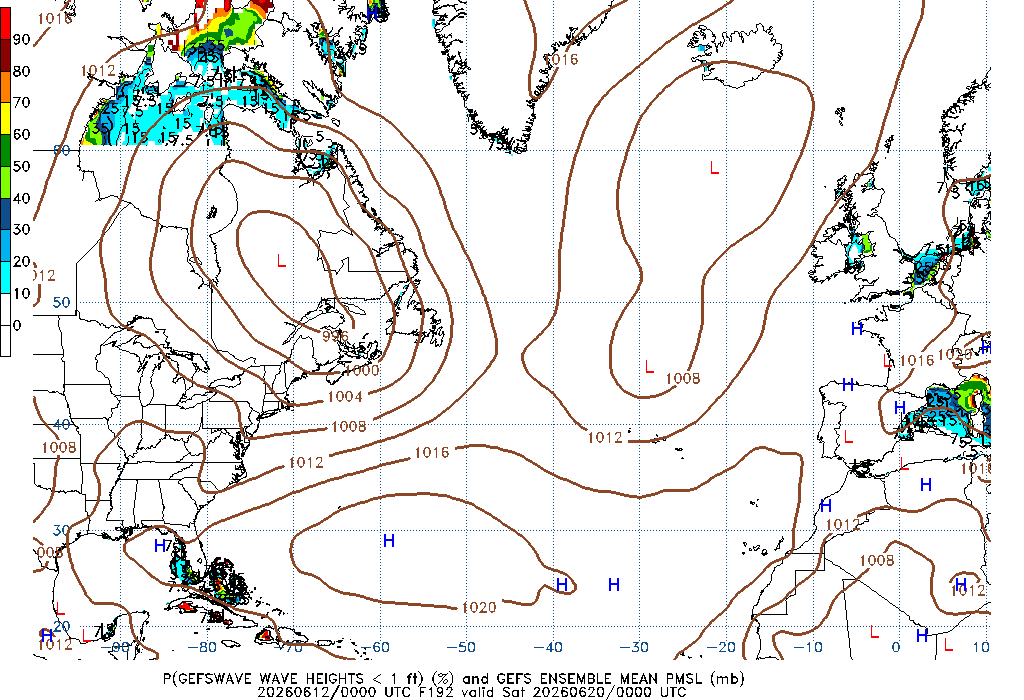192 Hour Wave Height less than 1ft 198 Hour Wave Height less than 1ft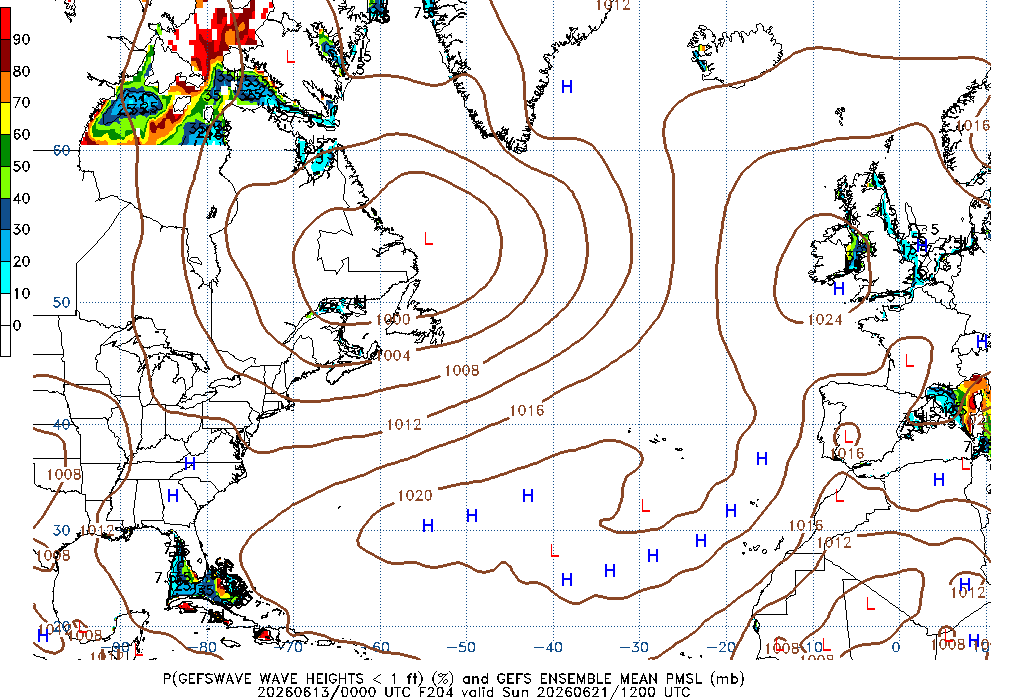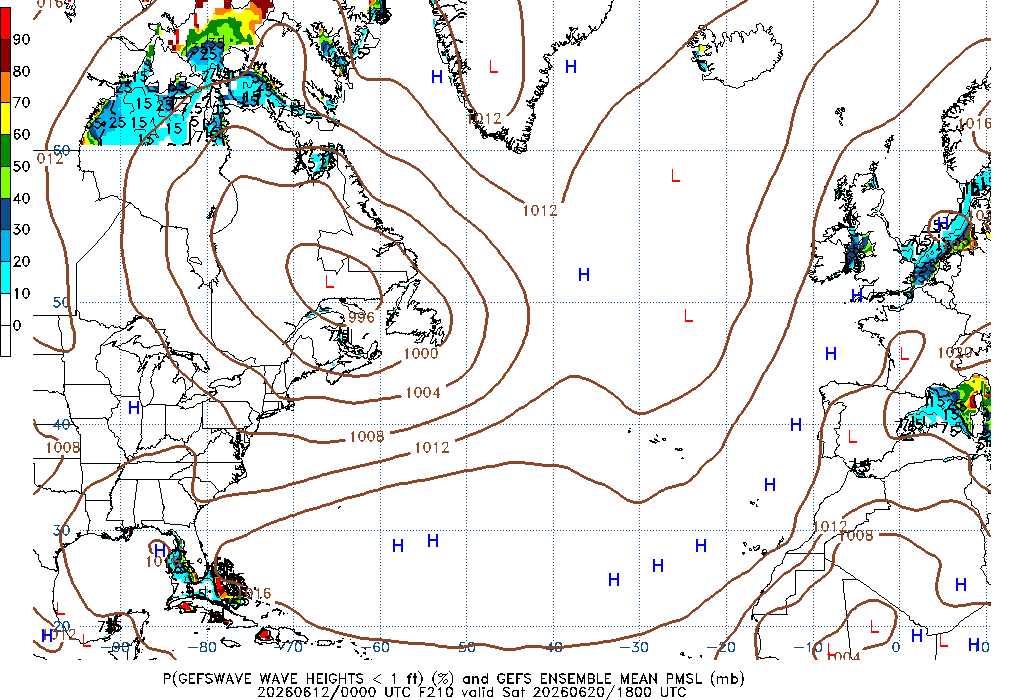204 Hour Wave Height less than 1ft 210 Hour Wave Height less than 1ft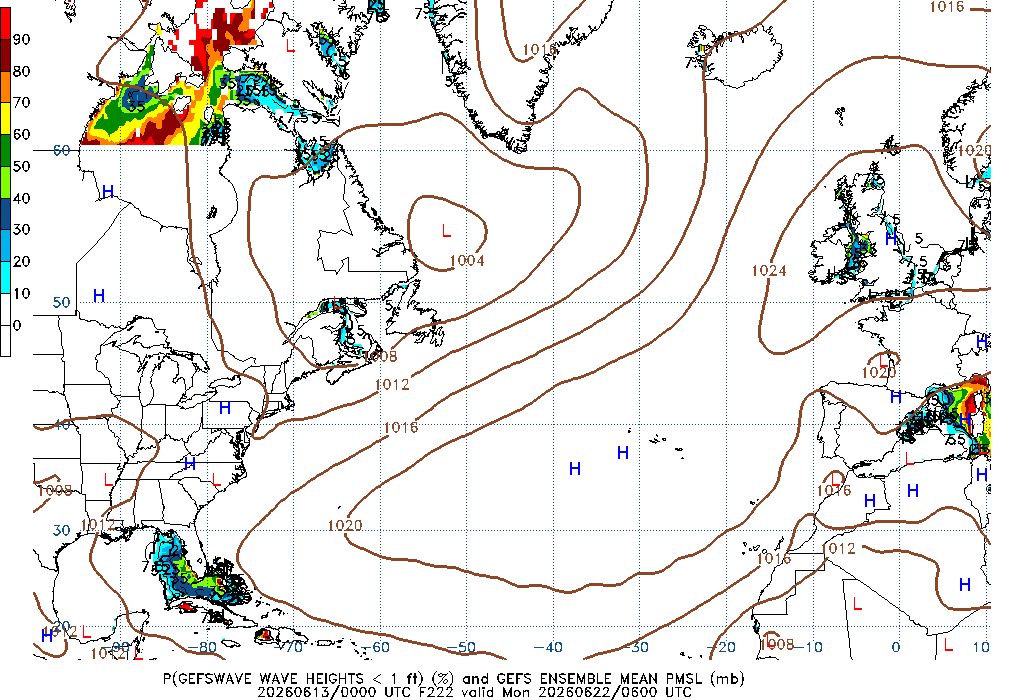216 Hour Wave Height less than 1ft 222 Hour Wave Height less than 1ft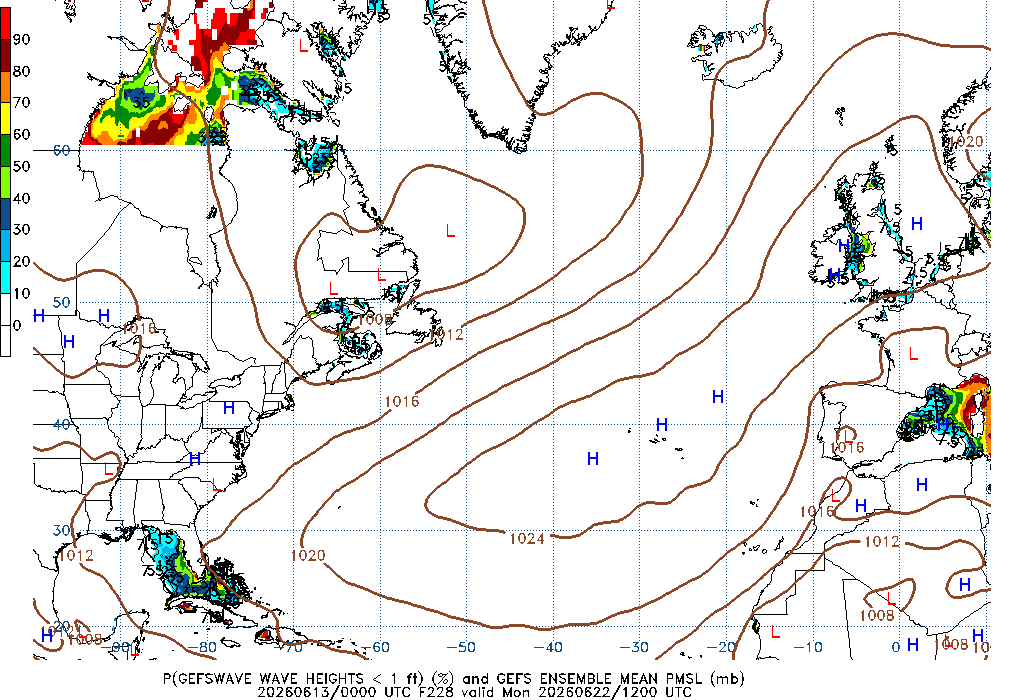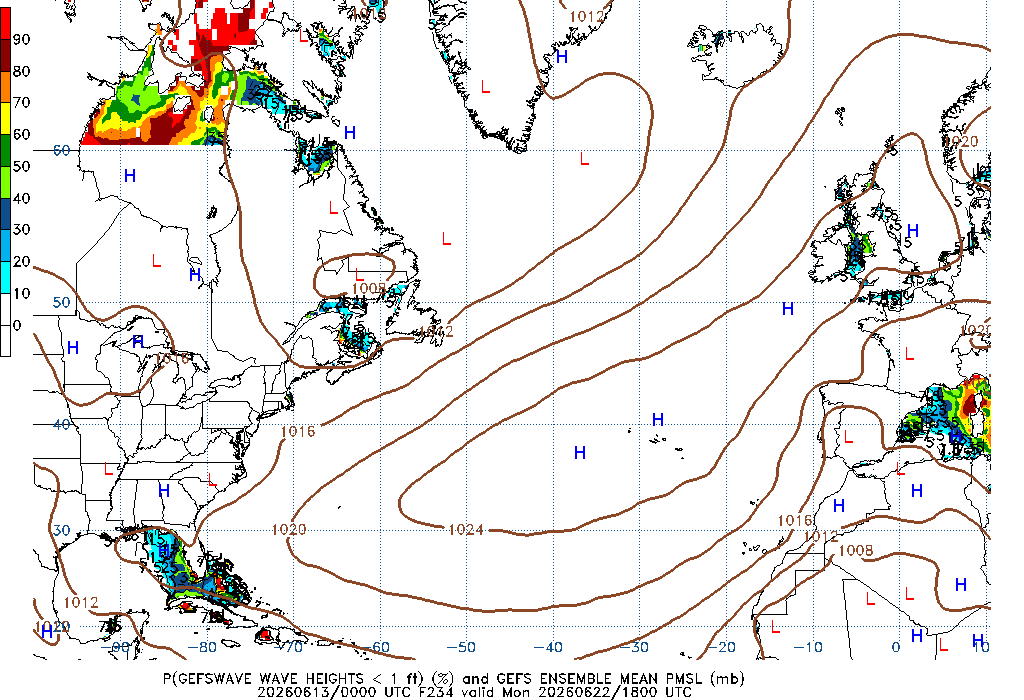228 Hour Wave Height less than 1ft 234 Hour Wave Height less than 1ft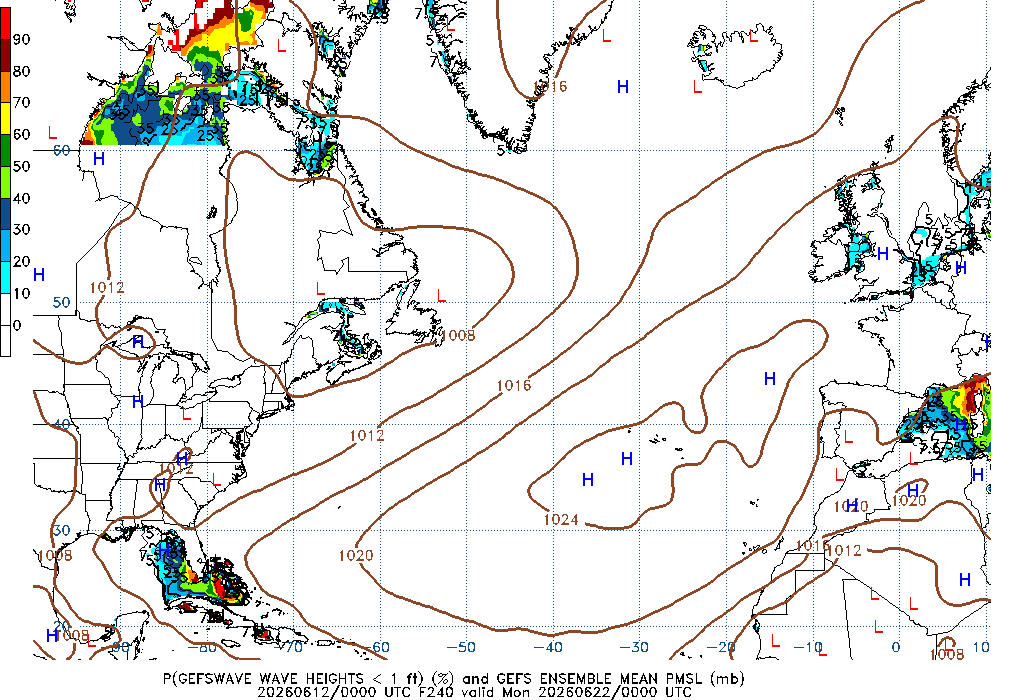240 Hour Wave Height less than 1ft

Notes on images above:

• The likelihood of exceedance is shown as a probability of 0 to 100 of waves heights (significant wave height) reaching or being greater than the chosen value.

• The 90th percentile represents the wave height value that 90% of the ensemble members are either less than or equal to (i.e., 18 feet).

• The 10th percentile is the wave height value that is represented by 10% of the contributing ensemble members.

• The mean is defined as the 50th percentile, that is, the wave height value represented reflects half the distribution is above and half is below this value.

• The maximum value is the highest value produced by any of the 31 ensemble members. It reflects the most severe conditions predicted by the ensemble prediction system.

The data are produced from the Global Ensemble Forecast System (GEFS) which is run every 6 hours and are created with 31 ensemble members.

Additional information for interpreting this data